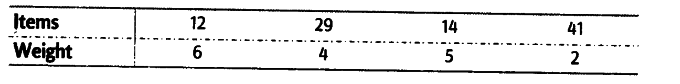# Calculate the weighted mean of the following distribution

Calculate the weighted mean of the following distribution